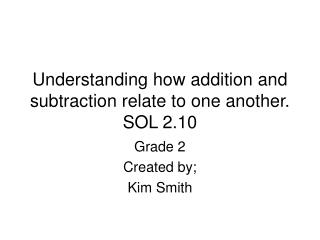DownloadDownload PresentationUnderstanding how addition and subtraction relate to one another. SOL 2.10

# Understanding how addition and subtraction relate to one another. SOL 2.10

Télécharger la présentation## Understanding how addition and subtraction relate to one another. SOL 2.10

- - - - - - - - - - - - - - - - - - - - - - - - - - - E N D - - - - - - - - - - - - - - - - - - - - - - - - - - -
##### Presentation Transcript

1. Understanding how addition and subtraction relate to one another.SOL 2.10 Grade 2 Created by; Kim Smith

2. 25 For each addition fact there is a related subtraction fact? • No • I don’t know • Who cares • Yes

3. 25 When determining a missing number do we have to add or subtract? • No • Yes • I don’t know • Maybe

4. 25 What are the missing numbers in this sentence?3+__=5 or __+2=5 • 3,1 • 2,3 • 1,3 • 5,5

5. 25 What are the missing numbers in this sentence?5-__=3 or 5-2=__ • 3,3 • 1,1 • 2,3 • 3,2

6. 25 What are the missing numbers in this sentence?6+__=10 or __+6=10 • 3,3 • 2,2 • 4,4 • 6,6

7. 25 What are the missing numbers in this sentence?8-__=2 or 8-6=___ • 6,2 • 3,1 • 8,8 • 8,2

8. 25 What are the missing numbers in this sentence?5-__=1 or 4+1=__ • 4,5 • 3,2 • 3,3 • 4,4

9. 25 What are the related facts for this addition problem?3 + 4 =7 • 7-2=5 and 7-5=2 • 7-3=4 and 7-4=3 • 7-6=1 and 7-1=6 • 3-4=7 and 4-3=7

10. 25 What are the related facts for this addition problem?6+5=11 • 11-6=6, 11+5=16 • 11-5=6, 11-6=5 • 11+11=22, 11+22=33 • 11-5=6, 11-6=6

11. 25 What are the related facts for this subtraction problem?15-6=9 • 9+6=15, 15-9=6 • 15-6=9, 15+6=21 • 15-9=6, 15-6=9 • 15+15=6, 15+15=30

12. 25 What are fact families? • When all the numbers are related • When all the numbers are different • When you only use addition • When you only use subtraction

13. 25 What are the fact families for10+3? • 10+3=13,3+10=13, 13-10=3,13-3=10 • 10+3=10,10+3=10,10-3=7, 10-7=3